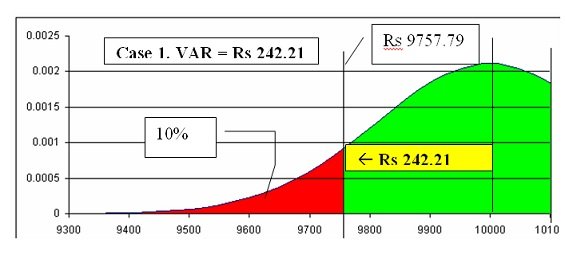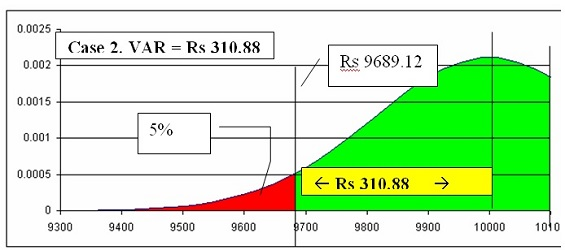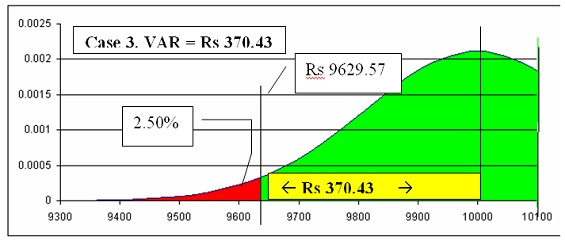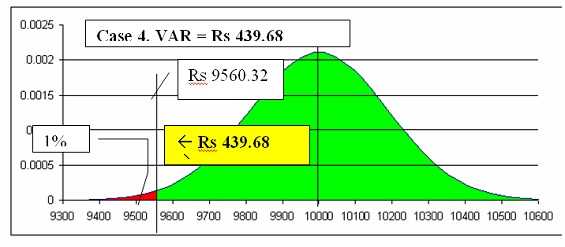# Value At Risk (VaR): Methodology for Measuring Financial Risk

CA Srikant AgarwalIn everyday life all of us are taking some amount of risk. Similarly a business also contains risk. Risk is the unexpected variability of asset prices and / or earnings. So risk is basically volatility and not necessarily loss.

There are major two sources of risk

2) Financial Risk

Business risk arises from the business decisions and business environment. Fall in demand, product development; capital budgeting decisions etc are the example of business risk.

Financial risk is the risk that arises from financial market activities such as currency exchange rate fluctuation, Interest rate risk etc. Financial risk can be segregated into four parts:

1) Market Risk (It arises due to volatility of prices in the market)

2) Liquidity Risk (It arises due to non-settlement of trades at fair prices)

3) Credit Risk (It arises due to possibility of default by counterparty)

4) Operational Risk (It arises due to failed internal processes, people, system or external events such as flood, earthquake etc)

In this article we will mainly emphasis upon financial risk and its measurement technique.

Everyone wants to minimize the amount of risk by taking various measures such as hedging, insurance, futures, forwards, options etc. Many times the risks are not insured and assumed by the firm itself (depending upon the amount of risk involved and capacity of the firm to bear the risk).

Before we try to hedge the risk, we need to measure risk as the strategy to hedge will depend upon amount of risk involved. If the amount of risk is within the capacity of the firm to assume, then the firm may not go for hedging and if the amount of risk is beyond the capacity of the firm to assume, then the firm should go for hedging the risk.

Each institution needs to measure the amount of risk its portfolio (comprising of stock, bonds, currencies and derivatives) may incur in the course of a day, week, month, or year.

There are two approaches of measuring the risk:

1) Traditional approaches: It includes calculation of standard deviation and beta of the portfolio. These methods of calculating risk are very complex and mainly depend on the historical data that generally shown to be weak during crisis.

2) Modern Approach: Value at Risk (VaR). It is in sharp contrast to traditional approaches and is developed to overcome the deficiency of traditional measure of risk.

Value at Risk (VaR)

Value at Risk means the maximum amount of loss a firm may incur over a period of time (a day, week, month or year) at a certain specified level of confidence (say 99%, 95%, 90% etc).

Example: If we say that 1 day VaR (Value at Risk) at 95% confidence level is Rs 100 Lacs. It implies that there is 5% chance that we may lose more than Rs 100 Lacs over a period of 1 day. Again it mean that there is a 95% chance that the amount of loss will be within Rs 100 Lacs over a period of 1 day.

The VaR is calculated by assuming normal distribution of statistics and can be calculated by applying following formula:

Note: The z value of normal distribution can be calculated by using the formula in Excel “=NORMSINV (% of significance)”. For e.g. NORMSINV(10%) = -1.282, NORMSINV(5%) = -1.645 and so on.

The calculation of VaR (Value at Risk) can be explained with the help of following illustration:

Illustration: Suppose that an investor’s portfolio consists entirely of Rs 10,000 worth of IBM stock. If the daily standard deviation of stock is 1.89% then what will be the Value at Risk at 90%, 95%, 97.50% and 99% confidence level over a period of 1 day.

###### (439.68)

In case 1, it shows that at 90% confidence level, VaR is Rs 242.21 i.e. there is 90% chance that we may lose up to Rs 242.21 over a period of 1 day and there is 10% chance that we may lose beyond Rs 242.21 over a period of 1 day.

Similarly in case 4 it shows that there is 99% chance that we may lose up to Rs 439.68 over a period of 1 day and 1% chance that we may lose beyond Rs 439.68 over a period of 1 day.

The above can also be shown with the help of normal distribution curve:It is apparent that as we increase the confidence level the value at risk increases. This is because of the fact that we want to be more confident about our calculation and hence maximum loss amount increases accordingly.

So if a firm finds that it may bear the loss up to Rs 439.68 (with 99% confidence level) then it may not go for hedging and if it is beyond tolerable limit then it may go for hedging in the form of forward, futures, options or other strategy to mitigate such loss.

In the similar manner we may calculate VaR for different time period by recalculating Standard Deviation for such period and may calculate VaR for more than one asset as well.

The above model of Value at Risk is subject to the limitation of GIGO (Garbage in garbage out) i.e. if the inputs w.r.t standard deviation , degree of confidence etc are incorrect then outcome will also be incorrect. Apart from this, it also ignores the worst case scenario as it assumes normal distribution.

As of now VaR (value at Risk) is one of the major tool for measuring risk and is widely used by all the banks and financial institution for measuring financial risk. Though it can well be applied by other organization in respect of their financial assets.